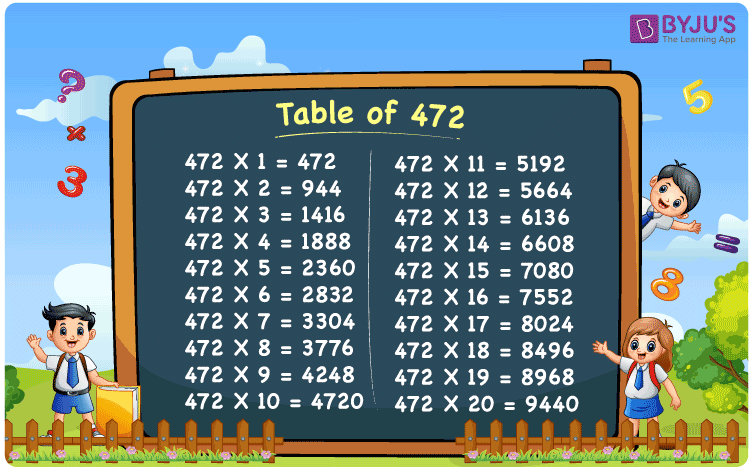Checkout JEE MAINS 2022 Question Paper Analysis : Checkout JEE MAINS 2022 Question Paper Analysis :

# Table of 472

The Multiplication Table for 472 is nothing more than the repetitive addition of the number 472. 472 is an even number that is divisible by 2. Note that 472 is also divisible by 4. Observe that all the multiples of 472 are also divisible by 4. You can read more on Divisibility rules.

## Table of 472 Chart## What is the 472 Times Table?

In the table of 472, we are repeatedly adding 472 multiple times. And the table below gives a repetitive addition 10 times for the number 472.

 472 × 1 = 472 472 472 × 2 = 944 472 + 472 = 944 472 × 3 = 1416 472 + 472 + 472 = 1416 472 × 4 = 1888 472 + 472 + 472 + 472 = 1888 472 × 5 = 2360 472 + 472 + 472 + 472 + 472 = 2360 472 × 6 = 2832 472 + 472 + 472 + 472 + 472 + 472 = 2832 472 × 7 = 3304 472 + 472 + 472 + 472 + 472 + 472 + 472 = 3304 472 × 8 = 3776 472 + 472 + 472 + 472 + 472 + 472 + 472 + 472 = 3776 472 × 9 = 4248 472 + 472 + 472 + 472 + 472 + 472 + 472 + 472 + 472 = 4248 472 × 10 = 4720 472 + 472 + 472 + 472 + 472 + 472 + 472 + 472 + 472 + 472 = 4720

## Multiplication Table of 472

Given below is the multiplication table of 472 being done 20 times. The below table helps you with multiplication problems that require the tables of 472.

 472 × 1 = 472 472 × 2 = 944 472 × 3 = 1416 472 × 4 = 1888 472 × 5 = 2360 472 × 6 = 2832 472 × 7 = 3304 472 × 8 = 3776 472 × 9 = 4248 472 × 10 = 4720 472 × 11 = 5192 472 × 12 = 5664 472 × 13 = 6136 472 × 14 = 6608 472 × 15 = 7080 472 × 16 = 7552 472 × 17 = 8024 472 × 18 = 8496 472 × 19 = 8968 472 × 20 = 9440

## Solved Example on Table of 472

Example:

Meera bought a few books at the cost of Rs 7080 online, and a few books at Rs 7552 from a shop. If each book costs 472, how many books did she buy in total?

Solution: Online 15 books , shop 16 books, total 31 books.

Online: Rs 7080, and each book costs Rs 472. Therefore 7080 / 472 = 15 books.

From Shop. Rs 7552, each book costs 474. Therefore 7552 / 472 = 16 books.

Total books = 15 + 16 = 31 books.

## Frequently Asked Questions on Table of 472

### Question 1: What is 24 times 472?

Answer: 24 times 472 is 11328.

### Question 2: Is 472 a multiple of 72?

Answer: No. 472 is not a multiple of 72.

### Question 3: What are the prime factors of 472?

Answer: The prime factors of 472 are 2, and 59.